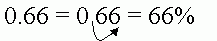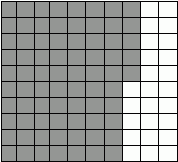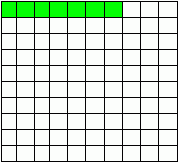Email us to get an instant 20% discount on highly effective K-12 Math & English kwizNET Programs!

#### Online Quiz (WorksheetABCD)

Questions Per Quiz = 2 4 6 8 10

### Grade 6 - Mathematics8.1 Percents

 Percent (or percentage) means "out of 100." We use the percent symbol (%) as a way to write a fraction with a common denominator of 100. For example, instead of saying "50 out of every 100 students are girls," we say "50% of students are girls." Example: 50% is shown as a partial shading of a grid as:A percent can always be written as a decimal, and a decimal can be written as a percent, by moving the decimal point two places to the right like this:Finding the Percent of a Number: Example: Find 50% of 20. Multiply the number by the percent (e.g. 20 x 50 = 1000) Divide the answer by 100 (Move decimal point two places to the left) (e.g. 1000/100 = 10). If the answer is in a decimal point, round it to the desired precision (e.g. 59.16 may be rounded to the nearest whole number = 59) Directions: Find the percentage of the shaded area. Use % symbol in your answer -- 20%, 50%, etc. Also write at least 10 examples of your own.
 Q 1: What is the percentage of the shading on the grid shown below?Answer: Q 2: What is the percentage of the shading on the grid shown below?Answer: Q 3: What is the percentage of the shading on the grid shown below?Answer: Q 4: What is the percentage of the shading on the grid shown below?Answer: Question 5: This question is available to subscribers only! Question 6: This question is available to subscribers only!

#### Subscription to kwizNET Learning System offers the following benefits:

• Unrestricted access to grade appropriate lessons, quizzes, & printable worksheets
• Instant scoring of online quizzes
• Progress tracking and award certificates to keep your student motivated
• Unlimited practice with auto-generated 'WIZ MATH' quizzes